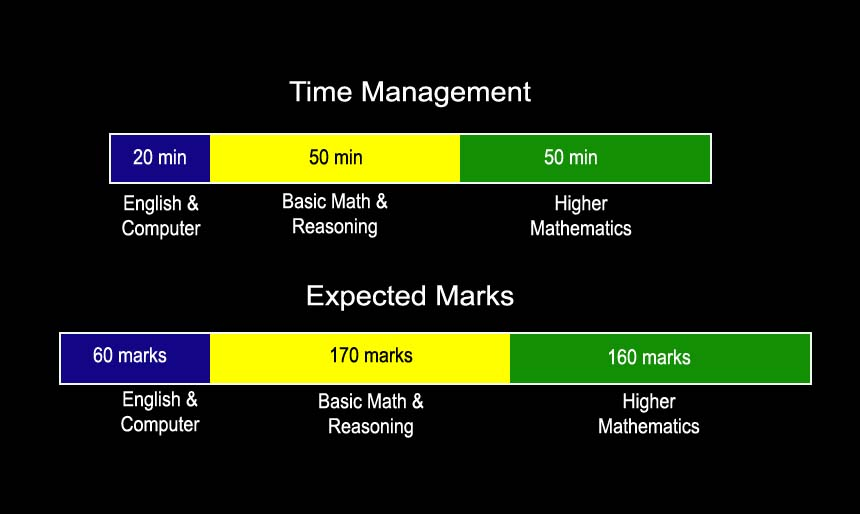## How to manage time in NIMCET PAPER to maximize score.

NIMCET is conducted to grant admission to students to 10 NIT’s and HCU (Hyderabad Central University). If a student gets rank above 400 (unreserved category), he can expect a seat in a decent NIT, (for reserved category, any rank even lower than this will suffice). First have a look at NIMCET paper pattern.

(i) 50 questions on higher mathematics, accounting for 600 marks, each question carries 12 marks.

(ii) 40 questions on reasoning and basic mathematics, accounting for 240 marks, each question carries 6 marks.

(iii) 10 questions on computers, accounting for 80 marks, each question carries 8 marks.

(iv) 20 questions on English, accounting for 80 marks, each question carries 4 marks.

Based on the level of last two years papers, a score of 350 – 400 will fetch you a good rank. Lets us see how an average student can manage this much in 120 minutes.

1. From the English and computer sections an average student can manage a score of 60 – 70. this means 6 to 7 questions from computer section and 4 to 5 questions from English section. Time taken in these sections must be around 20 minutes.

2. Reasoning and basic mathematics section is usually easy in NIMCET, from this section a student must target around 30 – 35 questions in approximately 40 to 50 minutes. Target marks from this section must be around 160 to 180.

3. Now we need approximately 160 more marks to secure a good rank, that means merely 15 questions from higher mathematics in the remaining time i.e. approximately 50 minutes.The above plan is for an average student who is not particularly good at higher mathematics, for the ones who are good at it, this target can go up to 30 – 40 questions of higher mathematics, that will put the student in the TOP 100 bracket.

Prepare some important topics of higher mathematics like Calculus, Coordinate Geometry, Vectors, progression, Probability, Permutation and combination etc. The more you practice, more questions you will be able to do, but the minimum target should be 15 questions.